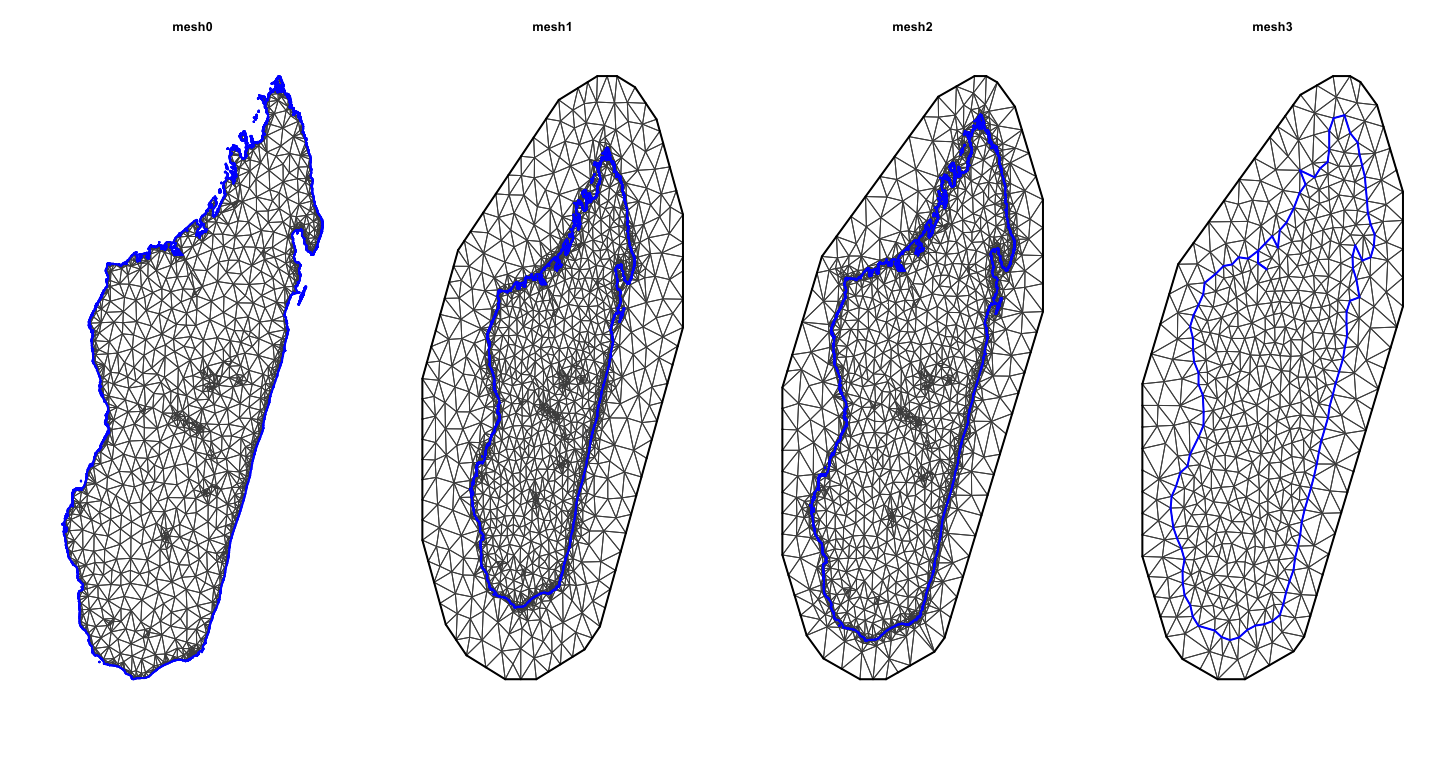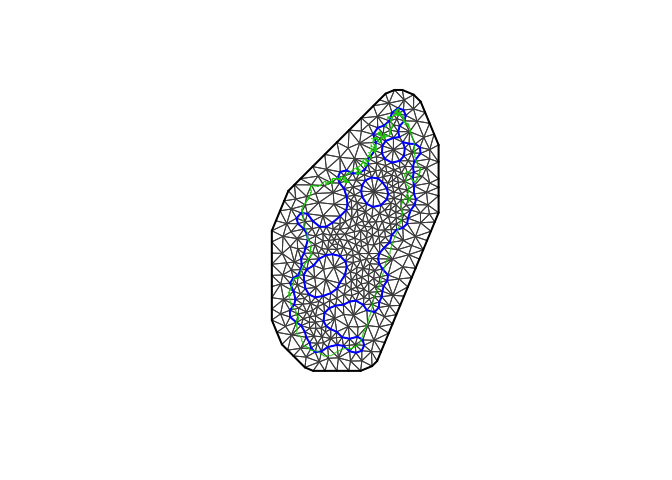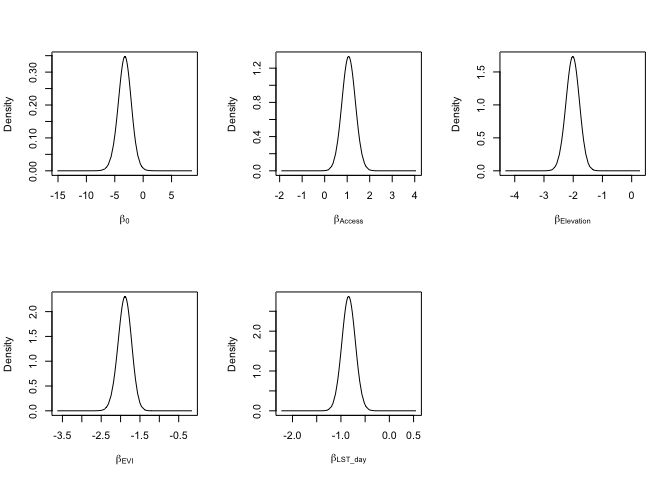The following presentation is to accompany this tutorial can be found HERE

## Overview

In this workshop we will be taking through an example of how to use the SPDE model using the R-INLA package. We will analyse parasite prevalence data from Madagascar. The topics we will cover include:

• How to create a mesh for the continuously indexed spatial effects
• Implementing the SPDE model in R-INLA
• Conducting simple model selection and best fit model
• Spatial prediction using R-INLA within and outside the fitting function (“inla”)
• Model validation

For a much more thorough description of R-INLA and the details underlying the SPDE models, please see Harvard & Rue 2015

### 1. Data

We recommend downloading the Rproject file from https://github.com/PunamA/BDI_INLA. First, download the zip file. Unzip and click the BDI_INLA.Rproj file. We recommend you to use the Intro_to_INLA_students.R Script. In case you want to get further details (or answers to any coding questions), we have provided you with our instructor script as well.

Malaria prevalence data: Open-access malaria data hosted by the Malaria Atlas Project accessed using the malariaAtlas R package. We are using Madagascar for this example

Covariate data: a suite of satellite imagery provided by MAP has been cleaned and processed for this tutorial. The data is available upon request from MAP For data cleaning and the preparation work please run the R-Script data_prep.R

For this tutorial, you do not need to run the preparation work script. The cleaned version of the data is available in input/MDG_clean.Rdata

load('input/MDG_clean.Rdata')

### 2. Model Description

Let $$Y_i$$ be the number of people tested positive (e.g. for malaria) which can be modelled as a binomial.

$Y_i \sim Binomial(p(s_i), N_i)$ where $$p(s_i)$$ is the probability of positive for location $$i$$ and $$N_i$$ is the number of examined. The linear predictor is expressed as linear combinations (thus, “linear”) of unknown parameters. The canonical link for the Binomial distribution is the logit function, which transforms probability [0,1] values into real values.

$logit(p(s_i)) = \beta_0 + X(s)\beta + \psi(s_i)$ where $$\beta_0$$ is the Intercept and $$\beta$$ is the covariate coefficient (slope). $$\psi(s_i)$$ is a gaussian field that can be approximated using a Gaussian Markov random field (GMRF) and can be represented as: $\psi(s_i)\sim N(0,\Sigma)$ where $$\Sigma$$ is a covariance matrix is determined by a spatial correlation function. In this case we will be using the Matern function:

$\frac{\sigma^2}{\Gamma(\lambda)2^{\lambda - 1}}(\kappa\parallel s_i - s_j\parallel)^\lambda K_\lambda(\kappa\parallel s_i - s_j\parallel)$ In this Bayesian context, we set priors for all parameters $\theta \sim \pi(\theta)$

### 3. Libraries needed and Installation

for installation please use

packages <- c("malariaAtlas", "raster", "sp", "tidyverse",
"lattice", "gridExtra", "devtools", "rlang")
if(length(setdiff(packages, rownames(installed.packages()))) > 0) {
install.packages(setdiff(packages, rownames(installed.packages()))) }

#For INLA!!
if(length(setdiff("INLA", rownames(installed.packages()))) > 0){
}

for this workshop we have included in the scripts the packages that need to be loaded:

library(INLA)
library(malariaAtlas)
library(raster)
library(sp)
library(tidyverse)
library(lattice)
library(gridExtra)

### 4. Creating the SPDE model

#### 4.1 The Mesh Construction

Disclaimer: there is no rule to determine the right size and spatial extension of the mesh. It is up to the analyst to set the mesh parameters, which vary from case to case. Models based on mesh with a large number of vertices are more computationally demanding and may not necessarily lead to better results than coarser mesh. Therefore, we recommend using a relatively coarse mesh during the preliminary phase of the analysis and use a finer grid only as final step, when the results of the analysis are satisfactory. To get more details on how to build a mesh, see Section 2.1. of Lindgren and Rue paper:

In order to create the mesh, we require the coordinated for the observed data points. In our analysis we also created the outline of the island boundary, but this isn’t necessary.

coords = cbind(MDG_pr_data$longitude, MDG_pr_data$latitude)
bdry <- inla.sp2segment(MDG_shp)
bdry$loc <- inla.mesh.map(bdry$loc)

Next we construct the mesh using coords. There are mutliple arguments that are required to build the mesh:

max.edge is the largest allowed triangle length; the lower the number the higher the resolution max.edge = c(inside the boundary triangle, outside the boundary triangle).

offset is defining how far you want to extend your domain (i.e. a secondary boundary box) for inner boundary and outer boundary; the offset goes with the max edge it should be in the same geographical unit as the max.edge

NOTE: including boundary argument makes the inner boundary value redundant. You can try by removing the boundary at this point and you’ll see a different inner and outer edge. Secondly, without the boundary, the mesh will be constructed based on a convex hull surrounding the observed points (i.e. coords). There are alternatives if you don’t want a non-convex hull.

cutoff can be used to avoid building too many small triangles around clustered data locations

mesh0 <- inla.mesh.2d(loc = coords, boundary = bdry, max.edge=c(0.5))
mesh1 <- inla.mesh.2d(loc = coords, boundary = bdry, max.edge=c(0.5, 1))
mesh2 <- inla.mesh.2d(loc = coords, boundary = bdry, max.edge=c(0.5, 1),
offset = c(0.5,1))
mesh3 <- inla.mesh.2d(loc = coords, boundary = bdry, max.edge=c(0.5,1),
offset = c(0.5, 1),
cutoff = 0.3)At this point, i would encourage you to try play with different values for each argument and observe the differences between the generated meshes.

the non-convex hull approach: we might want to use a mesh which is based on a non-convex hull to avoid adding many small triangles outside the domain of interest (more triangles = larger computation times), which can be done as follows:

non_convex_bdry <- inla.nonconvex.hull(coords, -0.03, -0.05, resolution = c(100, 100))
mesh4 <- inla.mesh.2d(boundary = non_convex_bdry, max.edge=c(0.5,1),
offset = c(0.5, 1),
cutoff = 0.3)The non-convex hull provides a mesh different from what we have seen previously. There are certain situations where it can be useful; for example when one wishes to model separate islands from the mainland. For more information we recommend reading F Lindgren’s blog.

#### 4.2. The SPDE and A matrix

Spatial models can be thought of as multivariate gaussian models; the correlation structure is determined by a spatial covariance function (modelled as a Matern covariance function), the full Gaussian field is approximated by a Gaussian Markov Random Field (GMRF). The GMRF approximation is computationally intense, which is efficiently performed in the R-INLA using the SPDE model. There are two components that are required to map the GMRF into the SPDE form.

1. the A matrix maps the Gaussian Markov Random Field (GMRF) from the mesh nodes to the n observation location. This is represented by a matrix with the number of observations as column and the number of nodes as rows.
A<-inla.spde.make.A(mesh=mesh3,loc=as.matrix(coords));dim(A)
##  357 557

Next, we create the spatial structure (SPDE object). The SPDE object is approximate at the mesh node. We use alpha=2 (fixed here, other values are not tested).

spde <- inla.spde2.matern(mesh3, alpha=2)

Lastly, we create all the required indexes for the SPDE model including naming the spatial field as “spatial.field”, which facilitates the extraction of the estimated values of the spatial field after the fitting process.

iset <- inla.spde.make.index(name = "spatial.field", spde$n.spde) #### 4.3. the INLA stack Since the covariates already are evaluated at the observation locations, we only want to apply the A matrix to the spatial effect and not the fixed effects. It is difficult to do this manually, but we can use the inla.stack function. Think of it as creating a list of items you require to build a model. The three main inla.stack() arguments are a vector list with the data (data), a list of projector matrices (each related to one block effect, A) and the list of effects (effects). Optionally, a label can be assigned to the data stack (using argument tag). stk <- inla.stack(data=list(y=MDG_pr_data$positive, n=MDG_pr_data$examined), #the response A=list(A,1), #the A matrix; the 1 is included to make the list(covariates) effects=list(c(list(Intercept=1), #the Intercept iset), #the spatial index #the covariates list(Elevation = MDG_pr_data$Elevation,
Access=MDG_pr_data$Access, LST_day = MDG_pr_data$LST_day,
Rain = MDG_pr_data$Rain, EVI = MDG_pr_data$EVI)
),

#this is a quick name so you can call upon easily
tag='dat')

Two projector matrices are needed A = list(A,1): the projector matrix for the latent field and a matrix that is a one-to-one map of the covariate. The latter matrix can simply be a constant rather than a diagonal matrix.

#### 4.4. Model Fitting

The first step before fitting the model is to express the linear predictor (see Section 2). For the sake of simplicity, we use one covariate only (Elevation).

$logit(p(s_i)) = \beta_0 + \beta_1Elevation + \psi(s_i)$

which translates to:

formula0<-y ~ -1 + Intercept + Elevation + f(spatial.field, model=spde) 

Note that to include the default ‘Intercept’ you can use +1. Some people prefer to not do this so they use -1 to exclude it and modify the data stack to include an intercept value in the effects list (just like us).

Once the formula is created we can fit the model

model0<-inla(formula0, #the formula
data=inla.stack.data(stk,spde=spde),  #the data stack
family= 'binomial',   #which family the data comes from
Ntrials = n,      #this is specific to binomial as we need to tell it the number of examined
control.predictor=list(A=inla.stack.A(stk),compute=TRUE),  #compute gives you the marginals of the linear predictor
control.compute = list(dic = TRUE, waic = TRUE, config = TRUE), #model diagnostics and config = TRUE gives you the GMRF
verbose = FALSE) #can include verbose=TRUE to see the log of the model runs

#### 4.5 INLA results

The results of the fitting process will be saved in your INLA object, here defined as model0. The object contains a lot of elements (51 elements). Here, we extract only the posterior distribution summaries of the parameters of interest: the fixed effects (intercept and Elevation) and hyper parameters (spatial field parameters).

model0$summary.fix ## mean sd 0.025quant 0.5quant 0.975quant mode ## Intercept -4.639712 1.398230 -7.459463 -4.621897 -1.917936 -4.585123 ## Elevation -1.734630 0.222806 -2.176097 -1.733239 -1.301208 -1.730431 ## kld ## Intercept 3.835996e-05 ## Elevation 4.059991e-06 model0$summary.hyperpar
##                               mean        sd 0.025quant  0.5quant
## Theta1 for spatial.field -6.262023 0.5248843  -7.417536 -6.205701
## Theta2 for spatial.field  2.218756 0.3486590   1.634934  2.182154
##                          0.975quant      mode
## Theta1 for spatial.field  -5.377439 -5.997638
## Theta2 for spatial.field   2.985948  2.045210

The hyperparameters $$\theta_1$$ is the $$log(\tau)$$ and $$\theta_2$$ is the $$log(\kappa)$$ from the SPDE framework. Briefly, the SPDE that represents the GMRF is

$$(\kappa^2 - \Delta)^{\alpha/2}(\tau \xi(s_i)) = W(s_i)$$.

Where $$\kappa$$ is the scale parameter and $$\tau$$ controls the variance, and $$W(s)$$ is the Gaussian Process. The posterior distribution of the hyperparameters $$\theta_1$$ and $$\theta_2$$ are difficult to interpret. We can transform them into more interpretable quantities such as the range, and the variance. The range is of particular interest. It provides the distance value (in the unit of the point coordinates) above which spatial dependencies become negligible.:

model0.res<-inla.spde2.result(model0, 'spatial.field', spde, do.transf=TRUE)
model0.res$summary.log.range.nominal ## ID mean sd 0.025quant 0.5quant 0.975quant ## range.nominal.1 5 -1.179169 0.3485897 -1.943657 -1.142141 -0.5921885 ## mode kld ## range.nominal.1 -1.029063 0 model0.res$summary.log.variance.nominal
##                    ID     mean        sd 0.025quant 0.5quant 0.975quant
## variance.nominal.1  4 5.559004 0.3989684   4.879105 5.519107   6.431093
##                        mode kld
## variance.nominal.1 5.398491   0

the do.tranf = TRUE makes sure that marginals are calculated in the same scale as the data.

### 5. Model Selection

There is a rich literature on variable selection in the field of statistics. A discussion of this topic would go beyond the scope of this tutorial. However, we show some ways to select variables using R-INLA built-in functions. Mainly we will be focus on metrics that we can include in R-INLA using control.compute function. In this function, one can extract several metrics helpful to select variables, including DIC, CPO/PIT, and WAIC. In the following example, we will focus on WAIC, but one can similarly extract DIC and CPO/PIT metrics.

###model selection with WAIC (other criteria can be used)
for(i in 1:5){

f1 <- as.formula(paste0("y ~ -1 + Intercept + f(spatial.field, model=spde) + ", paste0(colnames(covs_df)[1:i], collapse = " + ")))

model1<-inla(f1, data=inla.stack.data(stk,spde=spde),family= 'binomial',
Ntrials = n,
control.predictor=list(A=inla.stack.A(stk),compute=TRUE),
control.compute = list(dic = TRUE, cpo=TRUE, waic = TRUE)) #verbose=TRUE,

model_selection <- if(i==1){rbind(c(model = paste(colnames(covs_df)[1:i]),waic = model1$waic$waic))}else{rbind(model_selection,c(model = paste(colnames(covs_df)[1:i],collapse = " + "),waic = model1$waic$waic))
}

}

model_selection
##      model                                       waic
## [1,] "Access"                                    "9662.06025999789"
## [2,] "Access + Elevation"                        "9579.5657566753"
## [3,] "Access + Elevation + EVI"                  "9488.19106054678"
## [4,] "Access + Elevation + EVI + LST_day"        "9469.04240648151"
## [5,] "Access + Elevation + EVI + LST_day + Rain" "9486.92920832687"

Which model would you choose? It seems that Model 4 has the lowest WAIC. Therefore, we select Model 4.

#refit for best model:
formula<-y ~ -1 + Intercept + f(spatial.field, model=spde) + Access + Elevation + EVI + LST_day

model1<-inla(formula, data=inla.stack.data(stk,spde=spde),family= 'binomial',
Ntrials = n,
control.predictor=list(A=inla.stack.A(stk),compute=TRUE),
control.compute = list(dic = TRUE, waic = TRUE, config = TRUE),
verbose = FALSE) 

At this point, try exploring model1 using the code for INLA results. Is there anything new or interesting?

Now let’s look at the results of our analysis. The posterior (marginal) distribution of the fixed and hyper parameters will provide key insight into the effect of the covariates and the structure of the spatial field, respectively.

##observe the plots for fixed parameters
par(mfrow=c(2,3))
plot(model1$marginals.fixed[], ty = "l", xlab = expression(beta), ylab = "Density") plot(model1$marginals.fixed[], ty = "l", xlab = expression(beta[Access]), ylab = "Density")
plot(model1$marginals.fixed[], ty = "l", xlab = expression(beta[Elevation]), ylab = "Density") plot(model1$marginals.fixed[], ty = "l", xlab = expression(beta[EVI]), ylab = "Density")
plot(model1$marginals.fixed[], ty = "l", xlab = expression(beta[LST_day]), ylab = "Density")#observe the plots for hyper parameters par(mfrow=c(1,3)) plot(model1.res$marginals.var[], ty = "l", xlab = expression(sigma[randomfield]^2), ylab = "Density")
plot(model1.res$marginals.kap[], type = "l", xlab = expression(kappa), ylab = "Density") plot(model1.res$marginals.range[], type = "l", xlab = "range nominal", ylab = "Density")